# The Reaction MechanismPage 1

#### WATCH ALL SLIDES

Slide 1## Reaction Mechanism

The reaction mechanism is the series of elementary steps by which a chemical reaction occurs.

The sum of the elementary steps must give the overall balanced equation for the reaction

The mechanism must agree with the experimentally determined rate law

Slide 2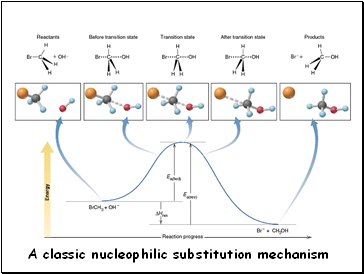A classic nucleophilic substitution mechanism

Slide 3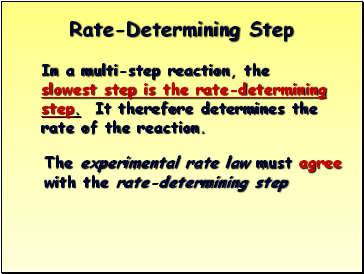## Rate-Determining Step

In a multi-step reaction, the slowest step is the rate-determining step. It therefore determines the rate of the reaction.

The experimental rate law must agree with the rate-determining step

Slide 4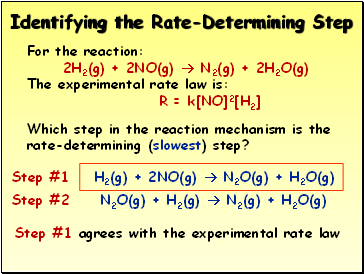## Identifying the Rate-Determining Step

For the reaction:

2H2(g) + 2NO(g)  N2(g) + 2H2O(g)

The experimental rate law is:

R = k[NO]2[H2]

Which step in the reaction mechanism is the rate-determining (slowest) step?

Step #1 H2(g) + 2NO(g)  N2O(g) + H2O(g)

Step #2 N2O(g) + H2(g)  N2(g) + H2O(g)

Step #1 agrees with the experimental rate law

Slide 5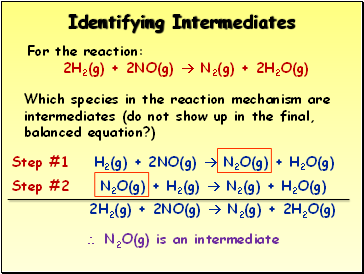## Identifying Intermediates

For the reaction:

2H2(g) + 2NO(g)  N2(g) + 2H2O(g)

Which species in the reaction mechanism are intermediates (do not show up in the final, balanced equation?)

Step #1 H2(g) + 2NO(g)  N2O(g) + H2O(g)

Step #2 N2O(g) + H2(g)  N2(g) + H2O(g)

2H2(g) + 2NO(g)  N2(g) + 2H2O(g)

 N2O(g) is an intermediate

Slide 6## Collision Model

Key Idea: Molecules must collide to react.

However, only a small fraction of collisions produces a reaction. Why?

Slide 7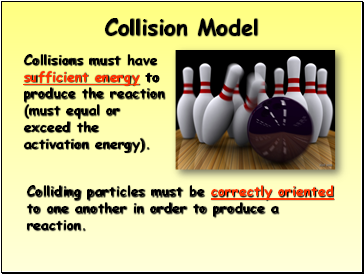Collision Model

Collisions must have sufficient energy to produce the reaction (must equal or exceed the activation energy).

Colliding particles must be correctly oriented to one another in order to produce a reaction.

Slide 8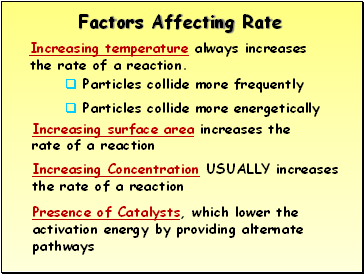## Factors Affecting Rate

Increasing temperature always increases the rate of a reaction.

Particles collide more frequently

Particles collide more energetically

Increasing surface area increases the rate of a reaction

Increasing Concentration USUALLY increases the rate of a reaction

Presence of Catalysts, which lower the activation energy by providing alternate pathways